# LOG#095. Group theory(XV).

The topic today in this group theory thread is “sixtors and representations of the Lorentz group”.

Consider the group of proper orthochronous Lorentz transformationsand the transformation law of the electromagnetic tensor. The components of this antisymmetric tensor can be transformed into a sixtor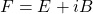orand we can easily write how the Lorentz group acts on this 6D vector ignoring the spacetime dependence of the field.

Under spatial rotations,transform separately in a well-known way giving you a reducible representation of the rotation subgroup in the Lorent orthochronous group. Remember that rotations are a subgroup of the Lorentz group, and it contains Lorentz boosts in addition to those rotations. In fact,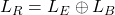in the space of sixtors and they are thus a reducible representation, a direct sum group representation. That is, rotations leave invariant subspaces formed by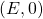andinvariant. However, these two subspaces mix up under Lorentz boosts! We have written before howtransform under general boosts but we can simplify it without loss of generalityandfor some matrices. So it really seems that the representation is “irreducible” under the whole group. But it is NOT true! Irreducibility does not hold if we ALLOW for COMPLEX numbers as coefficients for the sixtors/bivectors (so, it is “tricky” and incredible but true: you change the numbers and the reducibility or irreducibility character does change. That is a beautiful connection betweeen number theory and geometry/group theory). It is easy to observe that  using the Riemann-Silberstein vector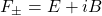and allowing complex coefficients under Lorent transformations, such thati.e., it transforms totally SEPARATELY from each other () under rotations and the restricted Lorentz group. However, what we do have is that using complex coefficients (complexification) in the representation space, the sixtor decomposes into 2 complex conjugate 3 dimensional representaions. These are irreducible already, so for rotations alonetransformations are complex orthogonal since if you write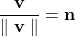withand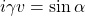. Be aware: here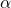is an imaginary angle. Moreover,transforms as follows from the following equation:Remark: Rotations in 4D are given by a unitary 4-vectorsuch asand the rotation matrix is given by the general formulaorIf you look at this rotation matrix, and you assign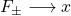with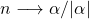, the above rotations are in fact the same transformations of the electric and magnetic parts of the sixtor! Thus the representation of the general orthochronous Lorentz group is secretly complex-orthogonal for electromagnetic fields (with complex coefficients)! We do know already that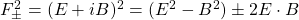are the electromagnetic main invariants. So, complex geometry is a powerful tool too in group theory! :). The real and the imaginary part of this invariant are also invariant. The matrices of 2 subrespresentations formed here belong to the complex orthogonal group. This group is a 3 dimensional from the complex viewpoint but it is 6 dimensional from the real viewpoint. The orthochronous Lorentz group is mapped homomorphically to this group, and since this map has to be real and analytic over the groupsuch that, as Lie groups,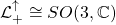. We can also use the complex rotation group in 3D to see that the 2 subrepresentations must be inequivalent. Namely, pick one of them as the definition of the group representation. Then, it is complex analytic and its complex parameter provide any equivalent representation. Moreover, any other subrepresentation is complex conjugated and thus antiholomorphic (in the complex sense) in the complex parameters.

Generally, having a complex representation, i.e., a representation in a COMPLEX space or representation given by complex valued matrices, implies that we get a complex conjugated reprentation which can be equivalent to the original one OR NOT. BUT, if share with original representation the property of being reducible, irreducible or decomposable. Abstract linear algebra says that to any representation in complex vector spacesthere is always a complex conjugate representation in the complex conjugate vector space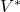. Mathematically, one ca consider representations in vector spaces over various NUMBER FIELDS. When the number field is extended or changed, irreducibility MAY change into reducibility and vice versa. We have seen that the real sixtor representation of the restricted Lorentz group is irreducible BUT it becomes reducible IF it is complexified! However, its defining representation by real 4-vectors remains irreducible under complexification. In Physics, reducibility is usually referred to the field of complex numbers, since it is generally more beautiful (it is algebraically closed for instance) and complex numbers ARE the ground field of representation spaces. Why is this so? There are two main reasons:

1st. Mathematical simplicity.is an algebraically closed filed and its representation theory is simpler than the one over the real numbers. Real representations are obtained by going backwards and “inverting” the complexification procedure. This process is sometimes called “getting the real forms” of the group from the complex representations.

2nd. Quantum Mechanics seems to prefer complex numbers (and Hilbert spaces) over real numbers or any other number field.

The importance of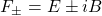is understood from the Maxwell equations as well. In vacuum, without sources or charges, the full Maxwell equations read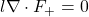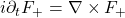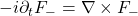These equations are Lorentz covariant and reducibility is essential there. It is important to note that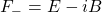implies that we can choose ONLY one of the components of the sixtor,or, or one single component of the sixtor is all that we need. If in the induction law there were a plus sign instead of a minus sign, then both representations could be used simultaneously! Furthermore, Lorentz covariance would be lost! Then, the Maxwell equations in vacuum should satisfy a Schrödinger like equation due to complex linear superposition principle. That is, ifandare solutions then a complex solution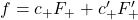with complex coefficients should also be a solution. This fact would imply invariance under the so-called duality transformation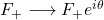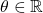However, it is not true due to the Nature of Maxwell equations and the (apparent) absence of isolated magnetic  charges and currents!

This site uses Akismet to reduce spam. Learn how your comment data is processed.